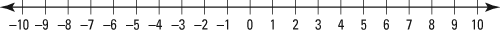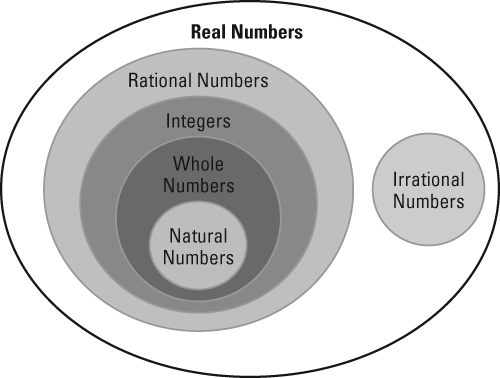##### TASC For DummiesThe TASC Math exam will likely contain questions where you'll need to be familiar with real numbers and the different number systems that constitute real numbers.

All real numbers can be classified as either rational or irrational, and the rational numbers can be further divided into subsets including natural numbers, whole numbers, and integers.

## Natural numbers

The natural numbers are commonly referred to as the counting numbers. The natural numbers start at 1 and proceed from there as if you were counting.

Natural numbers = {1, 2, 3, 4, . . .}

## Whole numbers

The whole numbers are a set that includes all the natural numbers and another element, zero. So, this set starts at 0 and counts up from there.

Whole numbers = {0, 1, 2, 3, . . .}

## Integers

The integers include both positive and negative versions of all the whole numbers. Think of this as a number line, with each tick mark being an integer, as shown here.

Integers = {. . . –3, –2, –1, 0, 1, 2, 3, . . .}The number line shows how positive and negative numbers extend to infinity in both directions.

## Rational numbers

Some people may try to generalize that all rational numbers are fractions. This isn't entirely true. A rational number is any number that can be written as an integer divided by an integer. So this includes traditional fractions, any terminating decimal, any repeating decimal, and any square root of a perfect square.

Some examples of rational numbers are

In addition, all integers, whole numbers, and natural numbers can be represented as a fraction:

## Irrational numbers

Rational numbers can be expressed as an integer divided by an integer; irrational numbers, on the other hand, are the set of numbers that can't be expressed in that form.

Some of the most common irrational numbers are the square roots of non-perfect squares. In addition, there are very famous irrational numbers, such as

The next figure illustrates how each of the classifications of numbers can be nested inside another. This means that to get the next layer out, new elements are added to create a larger set.Venn diagram illustrating how the number sets are contained in each other.

## Practice questions

1. The sum of 7/4 and pi is _____. A. always a rational number B. sometimes a rational number C. always an irrational number D. sometimes an irrational number
2. David launches a model rocket from a cliff that is 30 feet high off the ground. What is the possible domain for the height of the rocket with respect to the ground? A. all positive real numbers and zero B. all real numbers C. all integers D. all integers except zero